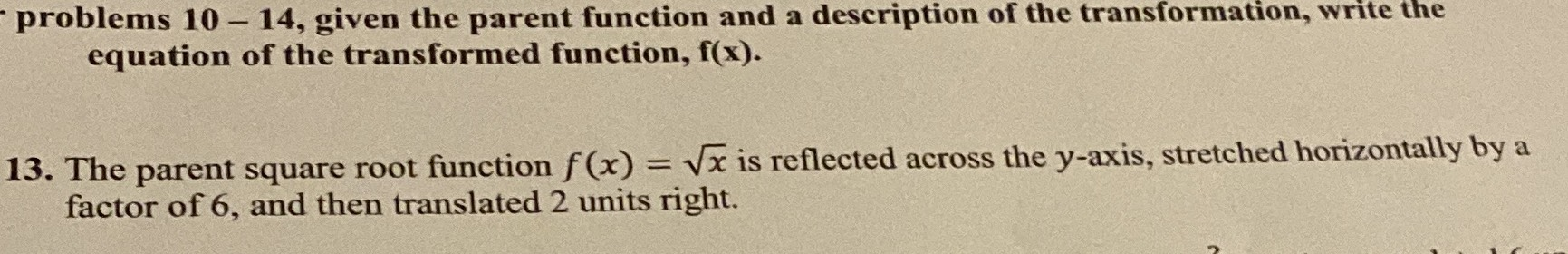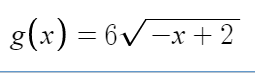### ¿Todavía tienes preguntas de matemáticas?

Pregunte a nuestros tutores expertos
Algebra
Pregunta

13. The parent square root function $$f ( x ) = \sqrt { x }$$ is reflected across the $$y$$ -axis, stretched horizontally by a factor of $$6$$ , and then translated $$2$$ units right.